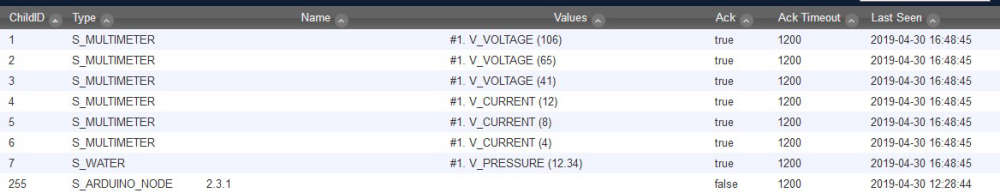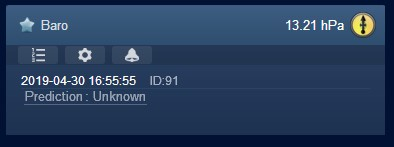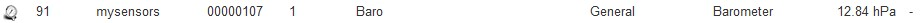# questioun using multiple sensors on domoticz

• Hi guys i building a multi sensor node , but i have one problem with a water pressure it keeps giving me a weather pressure , any one know what im doing wrong``````#define MY_NODE_ID 1
#define MY_DEBUG

#include <SPI.h>
#include <MySensors.h>

#define CHILD_ID_VOLT_1 1
#define CHILD_ID_VOLT_2 2
#define CHILD_ID_VOLT_3 3

#define CHILD_ID_CURRENT_1 4
#define CHILD_ID_CURRENT_2 5
#define CHILD_ID_CURRENT_3 6

#define CHILD_ID_PRESION 7

#define VOLT_SENSOR_ANALOG_PIN_1 0
#define VOLT_SENSOR_ANALOG_PIN_2 1
#define VOLT_SENSOR_ANALOG_PIN_3 2

#define CURRENT_SENSOR_ANALOG_PIN_1 3
#define CURRENT_SENSOR_ANALOG_PIN_2 4
#define CURRENT_SENSOR_ANALOG_PIN_3 5

#define PressPin      A6

unsigned long SLEEP_TIME = 20000; // Sleep time between reads (in milliseconds)

MyMessage msg(CHILD_ID_VOLT_1, V_VOLTAGE);
MyMessage msg2(CHILD_ID_VOLT_2, V_VOLTAGE);
MyMessage msg3(CHILD_ID_VOLT_3, V_VOLTAGE);

MyMessage msg4(CHILD_ID_CURRENT_1, V_CURRENT);
MyMessage msg5(CHILD_ID_CURRENT_2, V_CURRENT);
MyMessage msg6(CHILD_ID_CURRENT_3, V_CURRENT);

MyMessage pressureMsg(CHILD_ID_PRESION, V_PRESSURE);

float lastVolt1;
float lastVolt2;
float lastVolt3;

float lastCurrent1;
float lastCurrent2;
float lastCurrent3;

float lastPresion;

int pressure = 0;
float PSI = 0;
float PSI_CAL = 2.0;            // Calibration of sensor
int PSImsb = 0;
int PSIr = 0;

void before() {

}

void presentation()
{
sendSketchInfo("Pozo multi sensor", "1.0");  // Send the sketch version information to the gateway and Controller
present(CHILD_ID_VOLT_1, S_MULTIMETER);   // Register this device as power sensor
present(CHILD_ID_VOLT_2, S_MULTIMETER);   // Register this device as power sensor
present(CHILD_ID_VOLT_3, S_MULTIMETER);   // Register this device as power sensor

present(CHILD_ID_CURRENT_1, S_MULTIMETER);   // Register this device as power sensor
present(CHILD_ID_CURRENT_2, S_MULTIMETER);   // Register this device as power sensor
present(CHILD_ID_CURRENT_3, S_MULTIMETER);   // Register this device as power sensor

present(CHILD_ID_PRESION, S_WATER);   // Register this device as power sensor

}

void setup()
{

}

void loop()
{

float VoltLevel1 = map(Voltaje1,0,1023,0,500);
float VoltLevel2 = map(Voltaje2,0,1023,0,500);
float VoltLevel3 = map(Voltaje3,0,1023,0,500);

float CorrienteLevel1 = map(Corriente1,0,1023,0,200);
float CorrienteLevel2 = map(Corriente2,0,1023,0,200);
float CorrienteLevel3 = map(Corriente3,0,1023,0,200);

Serial.print("Voltaje L1: ");
Serial.println(VoltLevel1);
Serial.print("Voltaje L2: ");
Serial.println(VoltLevel2);
Serial.print("Voltaje L3: ");
Serial.println(VoltLevel3);

Serial.print("Corriente L1: ");
Serial.println(CorrienteLevel1);
Serial.print("Corriente L2: ");
Serial.println(CorrienteLevel2);
Serial.print("Corriente L3: ");
Serial.println(CorrienteLevel3);

//sensor de voltaje
if (VoltLevel1 != lastVolt1) {
send(msg.set(VoltLevel1, 1));
lastVolt1 = VoltLevel1;
}

if (VoltLevel2 != lastVolt2) {
send(msg2.set(VoltLevel2, 1));
lastVolt2 = VoltLevel2;
}
if (VoltLevel3 != lastVolt3) {
send(msg3.set(VoltLevel3, 1));
lastVolt3 = VoltLevel3;
}

//sensor de corriente
if (CorrienteLevel1 != lastCurrent1) {
send(msg4.set(CorrienteLevel1, 1));
lastCurrent1 = CorrienteLevel1;
}
if (CorrienteLevel2 != lastCurrent2) {
send(msg5.set(CorrienteLevel2, 1));
lastCurrent2 = CorrienteLevel2;
}
if (CorrienteLevel3 != lastCurrent3) {
send(msg6.set(CorrienteLevel3, 1));
lastCurrent3 = CorrienteLevel3;
}

/* ************************************************ */
wait(25);

/* • Output: 0.5V – 4.5V linear voltage output. 0 psi outputs 0.5V, 50 psi outputs 2.5V, 100 psi outputs 4.5V
0   psi = .33v after scalling 5.0v to 3.3v
50  psi = 1.65v
100 psi = 2.97v

3.3v/1024 = .0032266 volt per bit
*/

if (pressure < 106) pressure = 106;         // this is minimum of .5v
PSI = (pressure - 106 ) * .1246;            // where did we get this?? was .119904
PSI = PSI + PSI_CAL;                        // adjustment

PSImsb = PSI * 100;
PSIr = PSImsb % 100;

send(pressureMsg.set(PSI, 2));            // Send water pressure to gateway

Serial.print("Presion: ");
Serial.println(PSI);

wait(200);

// end of if (SLEEP_MODE || (cu

sleep(SLEEP_TIME);
}
``````any help will be very appreciated

• @fernando-alvarez-buylla S_WATER is a Volume or Flow subset from memory.

I've not yet got round to installing pressure sensors on the pumped supply, but had already settled on tracking numeric values of hydraulic pressure such as Bar or Metres typical of physical pressure meters, so V_DISTANCE and S_DISTANCE seem more appropriate and might be worth a try your end.

• S_WATER

Here you will see that with S_WATER you should use another V-TYPE:

• @alowhum Always been curious how Domoticz treats the V and S values, so tended to follow the MySensor API and had a few if unexpected moments and results along the way.
You implied that presentation of V_PRESSURE pre-determines an expectation of S_BARO irrespective of whatever further S_? statement follows.
Is this understanding correct?

6

1

14

1

2

2

7

1